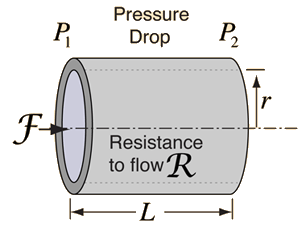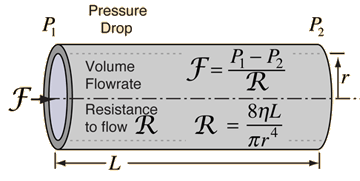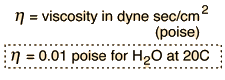# Poiseuille's LawIn the case of smooth flow (laminar flow), the volume flowrate is given by the pressure difference divided by the viscous resistance. This resistance depends linearly upon the viscosity and the length, but the fourth power dependence upon the radius is dramatically different. Poiseuille's law is found to be in reasonable agreement with experiment for uniform liquids (called Newtonian fluids) in cases where there is no appreciable turbulence.Calculation Example: changing flow parameters
Index

Poiseuille's law concepts

 HyperPhysics***** Mechanics ***** Fluids R Nave
Go Back

# Poiseuille's Law CalculationPoiseuille's law can be used to calculate volume flowrate only in the case of laminar flow.Any of the parameters below can be changed. When you have finished entering data, click on the quantity you wish to calculate in the formula above. The different parameters will not be forced to be consistent until you click on the quantity to calculate.
For pressure difference: x 10^ Pa = kPa
= atmos = mmHg = lb/in2 = cm water = inches water

applied to a tube of radius = cm = inches (corresponding to area =cm2 = in2)
and length = cm = inches,
for a fluid with viscosity = poise = x the viscosity of water,
the volume flowrate will be = cm3/s =in3/s=liters/min=ft3/min= U.S. gal/min.
Default values will be assigned to parameters to which you have not given values. You can change any of those default values as part of your exploration.
Index

Poiseuille's law concepts

 HyperPhysics***** Mechanics ***** Fluids R Nave
Go Back